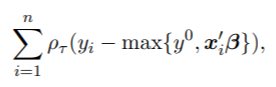# 贝叶斯_分位数_回归

Tobit RQ为描述非负因变量和协变量向量之间的关系提供了一种方法，可以被表述为因变量的数据未被完全观察到的分位数回归模型。关于Tobit 分位数回归模型有相当多的文献，我们可以参考Powell（1986）、Portnoy（2003）、Portnoy和Lin（2010）以及Kozumi和Kobayashi（2011）来了解概况。考虑一下这个模型。

Lasso回归、岭回归等正则化回归数学原理及R语言实例Yu和Stander（2007）提出了一种Tobit RQ的贝叶斯方法，使用ALD计算误差，并使用Metropolis-Hastings（MH）方法从其后验分布中抽取β。

# 真实数据实例

## 免疫球蛋白G数据

``rq(血清浓度~年龄, tau=0.5, runs=2000)``

``````
R> for (i in 1:5) {
+ taus=c(0.05, 0.25, 0.5, 0.75, 0.95)
+ rq(tau=taus[i],runs=500, burn=100)
+ abline(fit, col=i)
+ }
R>
R> for (i in 1:5) {
+ taus=c(0.05, 0.25, 0.5, 0.75, 0.95)
+ fit = rq(tau=taus[i],runs=500, burn=100)
+ }
``````

``plot(fit,"tracehist",D=c(1,2))``

# 前列腺癌数据

### 随时关注您喜欢的主题

``````R> x=as.matrix(x)
R> rq(y~x,tau = 0.5, method="BLqr", runs = 5000, burn = 1000, thin = 1)``````

``````plot(fit, plottype="trace", D=1:9)
plot(fit, plottype="hist", D=1:9)``````

# 下面的命令给出了τ=0.50时Tobit RQ的后验分布。

``````
rq(y~x,tau=0.5, methods="Btqr")``````

# 参考文献

Alhamzawi, R., K. Yu, and D. F. Benoit (2012). Bayesian adaptive lasso quantile regression. Statistical Modelling 12 (3), 279–297.

Brownlee, K. A. (1965). Statistical theory and methodology in science and engineering, Volume 150. Wiley New York.

Davino, C., M. Furno, and D. Vistocco (2013). Quantile regression: theory and applications. John Wiley & Sons.

Kaizong Ye拓端研究室（TRL）的研究员。

​非常感谢您阅读本文，如需帮助请联系我们！QQ在线咨询

15121130882

0571-63341498

## 关注有关新文章的微信公众号

This will close in 0 seconds